• 先求早开始时间：A是开始节点，所以A的早开始时间是0，并且早开始时间等于最晚开始时间。等得到图中红色的部分。 其他节点的早开始时间为以该节点作为弧头的所有有向弧的值+弧尾的值 的最大值，看例子就...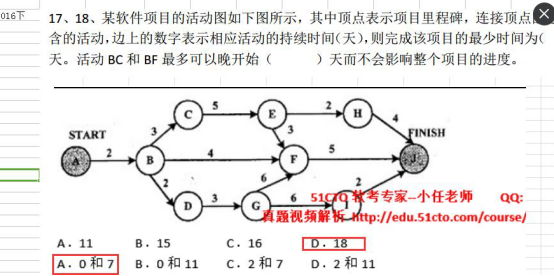题目如图所示，解法如下：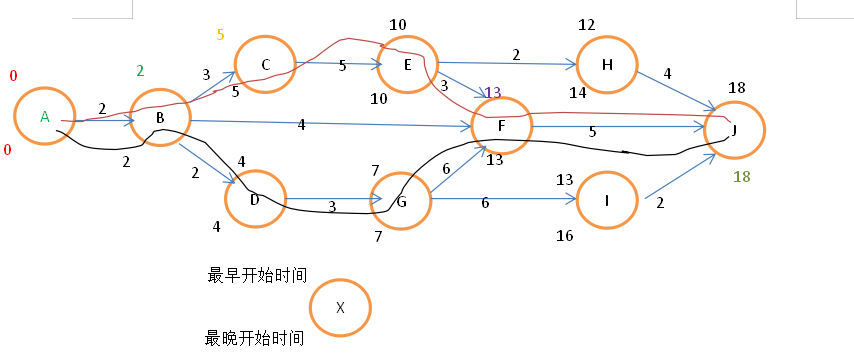方法：
先求最早开始时间：A是开始节点，所以A的最早开始时间是0，并且最早开始时间等于最晚开始时间。等得到图中红色的部分。
其他节点的最早开始时间为以该节点作为弧头的所有有向弧的值+弧尾的值 的最大值，看例子就明白了：
然后求其B的最早开始时间，由B作为弧头的有向弧只有<A,B>且值为2，A的最早开始时间为0，所以B的最早开始时间为0+2=2，得到绿色的结果。
求C的最早开始时间，以C为弧头的弧只有<B,C>且值为3，B的最早开始时间为2，所以C的最早开始时间为2+3=5，得到黄色结果。
同理D、G、E的最早开始时间如图所示。
求F的最早开始时间：以F为弧头的弧有<E,F> <B,F> <G,F> 所对应的值分别为 13 6 13,取MAX{13,6,13}得F的最早开始时间为13，得到紫色结果.
后面的都一样...
然后由于J是结束节点，所以最早开始时间与最晚开始时间一样，得到图中的 这个色结果。

接着求最晚开始时间：从后往前推。
先求I的最晚开始时间。应为以I为弧尾的只有弧<I,J>所以，I的最晚开始时间为J的最晚开始时间减去<I,J>的值 18-2=16
同理F H的最晚开始时间也可以得到。
求E的最晚开始时间： 以E为弧尾的弧有 <E,H> <E,F> H的最晚开始时间减去<E,H>的值为12   F的最晚开始时间减去<E,F>的值为13-3=10 ，取MIN{12,10}得到E的最晚开始时间为10
....
后面都一样了....

完成项目的最少时间就是结束节点的最早或最晚开始时间 18
两条关键路径都画在图中了
BC在关键路径上，所以一天也不能晚；
BF可以耽搁的时间为 F的最晚-B的最早-<B,F>的值，也就是 13-4-2=7

口诀：最早开始从前往后用加法看弧头最大，最晚开始从后往前用减法看弧尾最小

展开全文软件设计师
• 对于每个活动，列出它的前驱，并计算早开始时间、最晚开始时间和时差，然后确定出关键路径。 —— 《软件工程 第 4 版》中的原题 写文缘由 网上的文章大都是对于 “点” 求早开始时间和最晚开始时间。在我看来...
题目

下图是一个软件开发项目的活动图，对于图中每条边的数字表示完成这条边代表的活动的天数。例如，完成终止于里程碑E的活动需要 4 天时间。 对于每个活动，列出它的前驱，并计算最早开始时间、最晚开始时间和时差，然后确定出关键路径。 —— 《软件工程 第 4 版》中的原题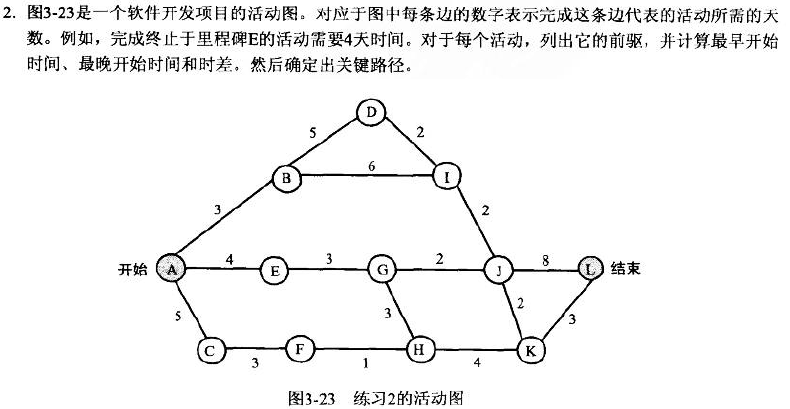写文缘由
网上的文章大都是对于 “点” 求最早开始时间和最晚开始时间。在我看来，是不准确的。
对于边的解法，有的写得又太复杂，还是自己写吧。顺便写个程序自动化一下，舒服~
误区在哪
需要注意的是，图中的点，并不代表活动，并不能说活动

A

A

用

3

3

天到达活动

B

B

，这是不准确的，图上的点应该理解为 “里程碑”。如果说到达 里程碑

I

I

的边有两条

D

→

I

D \rightarrow I

和

B

→

I

B \rightarrow I

，意思是有两个活动，完成后到达里程碑

I

I

，并不能说

I

I

是个活动，如果这么理解会在计算最晚开始时间时出现错误。
还有一点，时间轴从

1

1

开始算，即从点

A

A

出发时，时刻为

1

1

。有些解法是从

0

0

开始算的，本文从

1

1

开始算。
解法
正推求最早开始时间
公式：

ET

B

⋅

=

MAX

(

ET

A

B

+

w

A

B

)

\text{ET}_{B·} = \text{MAX}(\text{ET}_{AB} + w_{AB} )

已知条件：起点的最早开始时间直接为 1 倒推求最晚开始时间
公式：

LT

J

K

=

MIN

(

LT

K

⋅

−

w

J

K

)

\text{LT}_{JK} = \text{MIN}(\text{LT}_{K·} - w_{JK} )

已知条件：终点的最晚开始时间

LT

L

⋅

=

ET

L

⋅

\text{LT}_{L·}=\text{ET}_{L·}

（因为终点一定在关键路径上，关键路径上的点最早开始时间等于最晚开始时间）
解题示例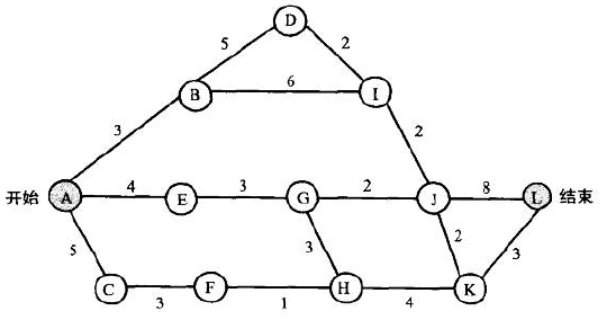解：
先求最早开始时间 (Earliest Time Start)：

A

A

是起点，所有由

A

A

出发的边的最早开始时间都为

1

1

。即

ET

A

B

=

ET

A

E

=

ET

A

C

=

ET

A

⋅

=

1

\text{ET}_{AB} = \text{ET}_{AE} = \text{ET}_{AC} =\text{ET}_{A·}= 1

来算

B

B

的最早开始时间，

ET

B

D

=

ET

B

I

=

ET

B

⋅

=

MAX

(

ET

A

B

+

w

A

B

)

=

4

\text{ET}_{BD} = \text{ET}_{BI} = \text{ET}_{B·} =\text{MAX}(\text{ET}_{AB}+w_{AB}) =4

。因为只有

A

A

才能到达

B

B

，所以 MAX 内只有一个值。来算

E

E

的最早开始时间，

ET

E

G

=

MAX

(

ET

A

E

+

w

A

E

)

=

5

\text{ET}_{EG} =\text{MAX}(\text{ET}_{AE}+w_{AE}) =5

。因为只有

A

A

才能到达

E

E

，所以 MAX 内只有一个值。来算

C

C

的最早开始时间，

ET

C

F

=

MAX

(

ET

A

C

+

w

A

C

)

=

6

\text{ET}_{CF} =\text{MAX}(\text{ET}_{AC}+w_{AC}) =6

。因为只有

A

A

才能到达

C

C

，所以 MAX 内只有一个值。接下来

D

、

G

、

F

D、G、F

同理，省去废话，结果是：

ET

D

I

=

MAX

(

ET

B

D

+

w

B

D

)

=

9

\text{ET}_{DI} =\text{MAX}(\text{ET}_{BD}+w_{BD}) =9

、

ET

G

J

=

ET

G

H

=

ET

G

⋅

=

MAX

(

ET

E

G

+

w

E

G

)

=

8

\text{ET}_{GJ} =\text{ET}_{GH}=\text{ET}_{G·} =\text{MAX}(\text{ET}_{EG}+w_{EG}) =8

、

ET

F

H

=

MAX

(

ET

C

F

+

w

C

F

)

=

9

\text{ET}_{FH} =\text{MAX}(\text{ET}_{CF}+w_{CF}) =9

看一下

I

I

，入度为

2

2

，

ET

I

J

=

MAX

(

ET

D

I

+

w

D

I

,

ET

B

I

+

w

B

I

)

=

MAX

(

11

,

10

)

=

11

\text{ET}_{IJ} =\text{MAX}(\text{ET}_{DI}+w_{DI}, \text{ET}_{BI}+w_{BI}) =\text{MAX}(11, 10) =11

，下面的同理

ET

H

K

=

MAX

(

ET

G

H

+

w

G

H

,

ET

F

H

+

w

F

H

)

=

MAX

(

11

,

10

)

=

11

\text{ET}_{HK} =\text{MAX}(\text{ET}_{GH}+w_{GH}, \text{ET}_{FH}+w_{FH}) =\text{MAX}(11, 10) =11

ET

J

L

=

ET

J

K

=

MAX

(

ET

I

J

+

w

I

J

,

ET

G

J

+

w

G

J

)

=

MAX

(

13

,

10

)

=

13

\text{ET}_{JL}=\text{ET}_{JK} =\text{MAX}(\text{ET}_{IJ}+w_{IJ}, \text{ET}_{GJ}+w_{GJ}) =\text{MAX}(13, 10) =13

ET

K

L

=

MAX

(

ET

J

K

+

w

J

K

,

ET

H

K

+

w

H

K

)

=

MAX

(

15

,

15

)

=

15

\text{ET}_{KL}=\text{MAX}(\text{ET}_{JK}+w_{JK}, \text{ET}_{HK}+w_{HK}) =\text{MAX}(15, 15) =15

ET

L

⋅

=

MAX

(

ET

J

L

+

w

J

L

,

ET

K

L

+

w

K

L

)

=

MAX

(

21

,

18

)

=

21

\text{ET}_{L·}=\text{MAX}(\text{ET}_{JL}+w_{JL}, \text{ET}_{KL}+w_{KL}) =\text{MAX}(21, 18) =21

自此，最早开始时间全部算完。
再求最晚开始时间 (Latest Time Start)：
从终点倒着推，

LT

J

L

=

MIN

(

LT

L

⋅

−

w

J

L

)

=

13

\text{LT}_{JL}=\text{MIN}(\text{LT}_{L·}-w_{JL}) =13

，

LT

K

L

=

MIN

(

LT

L

⋅

−

w

K

L

)

=

18

\text{LT}_{KL}=\text{MIN}(\text{LT}_{L·}-w_{KL}) =18

LT

J

K

=

MIN

(

LT

K

L

−

w

J

K

)

=

16

\text{LT}_{JK}=\text{MIN}(\text{LT}_{KL}-w_{JK}) =16

LT

I

J

=

MIN

(

LT

J

L

−

w

I

J

,

LT

J

K

−

w

I

J

)

=

11

\text{LT}_{IJ}=\text{MIN}(\text{LT}_{JL}-w_{IJ}, \text{LT}_{JK}-w_{IJ}) =11

LT

G

J

=

MIN

(

LT

J

L

−

w

G

J

,

LT

J

K

−

w

G

J

)

=

11

\text{LT}_{GJ}=\text{MIN}(\text{LT}_{JL}-w_{GJ}, \text{LT}_{JK}-w_{GJ}) =11

LT

H

K

=

MIN

(

LT

K

L

−

w

H

K

)

=

14

\text{LT}_{HK}=\text{MIN}(\text{LT}_{KL}-w_{HK}) =14

LT

D

I

=

MIN

(

LT

I

J

−

w

D

I

)

=

9

\text{LT}_{DI}=\text{MIN}(\text{LT}_{IJ}-w_{DI}) =9

LT

B

I

=

MIN

(

LT

I

J

−

w

B

I

)

=

5

\text{LT}_{BI}=\text{MIN}(\text{LT}_{IJ}-w_{BI}) =5

LT

G

H

=

MIN

(

LT

H

K

−

w

G

H

)

=

11

\text{LT}_{GH}=\text{MIN}(\text{LT}_{HK}-w_{GH}) =11

LT

B

D

=

MIN

(

LT

D

I

−

w

B

D

)

=

4

\text{LT}_{BD}=\text{MIN}(\text{LT}_{DI}-w_{BD}) =4

LT

E

G

=

MIN

(

LT

G

J

−

w

G

H

,

LT

G

H

−

w

E

G

)

=

8

\text{LT}_{EG}=\text{MIN}(\text{LT}_{GJ}-w_{GH}, \text{LT}_{GH}-w_{EG}) =8

LT

F

H

=

MIN

(

LT

H

K

−

w

F

H

)

=

13

\text{LT}_{FH}=\text{MIN}(\text{LT}_{HK}-w_{FH}) =13

LT

C

F

=

MIN

(

LT

F

H

−

w

C

F

)

=

10

\text{LT}_{CF}=\text{MIN}(\text{LT}_{FH}-w_{CF}) =10

LT

A

B

=

MIN

(

LT

B

D

−

w

A

B

,

LT

B

I

−

w

A

B

)

=

1

\text{LT}_{AB}=\text{MIN}(\text{LT}_{BD}-w_{AB},\text{LT}_{BI}-w_{AB}) =1

LT

A

E

=

MIN

(

LT

E

G

−

w

A

E

)

=

4

\text{LT}_{AE}=\text{MIN}(\text{LT}_{EG}-w_{AE}) =4

LT

A

C

=

MIN

(

LT

C

F

−

w

A

C

)

=

5

\text{LT}_{AC}=\text{MIN}(\text{LT}_{CF}-w_{AC}) =5

（上述过程，看似繁琐，但是考试计算时，在图中对应的边上边写边算，还是挺快的）
根据上述数据，列表如下（其中冗余时间等于最早最晚两者的差）：
活动前驱最早开始时间最晚开始时间时差（冗余时间）AB110BDAB440BIAB451DIAB,BD990IJAB,BD,DI,BI11110AE143EGAE583GJAE,EG8113JLAB,BD,BI,DI,IJ,AE,EG,GJ13130AC154CFAC6104FHAC,CF9134GHAE,EG8113HKAE,EG,GH,AC,CF,FH11143JKAB,BD,BI,DI,IJ,AE,EG,GJ13163KLAB,BD,BI,DI,IJ,AE,EG,GJ,JK,GH,AC,CF,FH,HK15183
由上述表格可知，

A

B

、

B

D

、

D

I

、

I

J

、

J

L

AB、BD、DI、IJ、JL

活动的时差为

0

0

，即为关键节点，因此关键路径为

A

→

B

→

D

→

I

→

J

→

L

=

20

A\rightarrow B\rightarrow D\rightarrow I\rightarrow J\rightarrow L=20

。
程序实现
诶，写个程序验证一下手算的正确与否吧。
#include <bits/stdc++.h>
using namespace std;
#define rep(i,s,t) for(int i=s;i<=t;i++)
const int maxn = 105;
const int INF = 0x3f3f3f3f;

int n, m, S, T;

struct Edge {
int u, v, w, ET, LT;
Edge(int _u,int _v,int _w,int _ET,int _LT):u(_u),v(_v),w(_w),ET(_ET),LT(_LT){}
};
vector<Edge*> G[maxn], GT[maxn], Edges; // 正图和反图

int calcET(Edge *e)
{
if (e->u == S)
return e->ET = 1;

for(Edge *ee : GT[e->u])
e->ET = max(e->ET, (ee->ET==-1?calcET(ee):ee->ET) + ee->w);

return e->ET;
}

int calcLT(Edge *e)
{
if (e->u == T)
return e->LT = e->ET;

for(Edge *ee : G[e->v])
e->LT = min(e->LT, (ee->LT==INF?calcLT(ee):ee->LT) - e->w);

return e->LT;
}

bool vis[maxn];
vector<int> path;
void dfs(int u)
{
if (u == T)
{
path.push_back(u);
for(int i=0;i<path.size();i++)
printf(i!=path.size()-1?"%c->":"%c\n", path[i]+'A'-1);
path.pop_back();
return;
}
vis[u] = true;
for (Edge *e : G[u])
if(!vis[e->v] && e->ET==e->LT)
{
path.push_back(u);
dfs(e->v);
path.pop_back();
}
vis[u] = false;
return;
}

char s;
int main()
{
freopen("out.txt", "w", stdout);
scanf("%d%d",&n,&m);
rep(i,1,m)
{
int u, v, w;
scanf("%s", s); u = s-'A'+1;
scanf("%s", s); v = s-'A'+1;
scanf("%d", &w);
Edge* e = new Edge(u, v, w, -1, INF);
G[u].push_back(e);
GT[v].push_back(e);
Edges.push_back(e);
}
// 默认 1 是起点, n 是终点，起点入度为 0，终点出度为 0，数据合法。不是的话得改造程序求个拓扑之类的。
S = 1;
T = n;
// 算 ET
G[T].push_back(new Edge(T, -1, 0, -1, INF));
calcET(G[T].back());
// 算 LT
calcLT(new Edge(-1, S, 0, -1, INF));
// 输出表
for(Edge *e : Edges)
printf("%c%c\t%d\t%d\t%d\n", e->u+'A'-1, e->v+'A'-1, e->ET, e->LT, e->LT-e->ET);
printf("%c.\t%d\t%d\t%d\n", G[T].back()->u+'A'-1, G[T].back()->ET, G[T].back()->LT, G[T].back()->LT-G[T].back()->ET);
// 求关键路径
dfs(S);
return 0;
}
/*
12 16
A B 3
A E 4
A C 5
B D 5
B I 6
E G 3
C F 3
D I 2
I J 2
G J 2
G H 3
F H 1
J L 8
J K 2
H K 4
K L 3

12 15
A B 2
B C 3
B F 4
B D 2
C E 5
D G 3
E H 2
E F 3
F I 5
G I 6
I J 2
I K 4
J L 1
K L 2
H L 3

12 17
A B 5
A E 3
A C 4
B D 6
B I 4
E G 4
C F 3
D I 3
I J 3
G I 2
G J 7
F G 6
F H 3
J L 9
H J 3
H K 6
K L 2

12 17
A B 5
A E 3
A C 4
B D 6
B I 4
E G 4
C F 3
D I 5
I J 4
G I 2
G J 7
F G 6
F H 3
J L 9
H J 3
H K 6
K L 2

12 17
A B 6
A E 10
A C 4
B D 6
B I 4
E G 4
C F 3
D I 5
F G 6
G I 2
G J 7
I J 4
F H 3
J L 9
H J 3
H K 6
K L 2

*/

尾巴
好的，感谢你看到这里，对文章有错误的地方欢迎指出，谢谢。 如果觉得本文写得不错，不妨点赞、评论、收藏、分享，你的三连是对我最大的支持！
我的 Github：zhangt2333’s Github 我的 CSDN：zhangt2333’s CSDN 我的 博客园：zhangt2333’s cnblog 我的 小书房：https://zhangt.top/
本文作者：zhangt2333 版权声明：本博客所有文章除特别声明外，均采用 CC BY-NC-SA 4.0 许可协议 。转载请注明出处！
展开全文• 2）一个活动的最晚开始几天不影响，或者一个活动可以耽搁的时间，是有公式计算的 3）关键路径为起点到终点权值加起来最大的路径 直接例题 首先，关键路径为最大（大）的总值，计算可以得到ABCEFJ=18,所以关键路径的...
1）一个点的最早/最晚时间是有公式或者技巧求出来的
2）一个活动的最晚开始几天不影响，或者一个活动可以耽搁的时间，是有公式计算的
3）关键路径为起点到终点权值加起来最大的路径
直接例题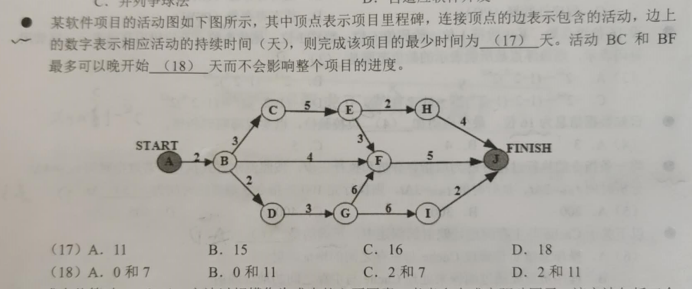首先，关键路径为最大（大）的总值，计算可以得到ABCEFJ=18,所以关键路径的值为18
一、一个点的最早开始时间：从起点到该点的最长（大）的值
A：起点，最早开始时间为0B：起点到B只有AB=2C：起点到C只有ABC=2+3=5D：起点到D只有ABD=2+2=4E：起点到E只有ABCE=2+3+5=10F：起点到F有ABCEF=13 ABF=6 ABDGF=13 取最大，其中ABCEF和ABDGF一样值，所以F的最早开始时间为13G：ABDG=7H：ABCEH=12I：ABDGI=13J：J有H，F，I 这3个点到J，其中ABCEHJ=12+4=16 0到F最大+<F,J>=13+5=18 0到I最大+<I,J>=13+2=15 取最大，所以J的最早开始时间为18
二、一个点的最晚开始时间：关键路径的值 - 终点到该点的最大的值
或者这样理解：关键路径的值 - 终点到该点的值，如果得到的有多个值，那么取最小
MIN { 关键路径的值 - 终点到该点的值 }
为了方便运算，求最晚的都是通过反方向来求的
J：0H：重点到H只有JH，18-4=14F：18-5=13I：18-2=16E：有JFE=8 JHE=6 有最晚开始时间MIN{ 18-8=10，18-6=12 }=10G：有JFG=11 JIG=8 最晚开始时间 MIN{ 18-11=7，18-8=10}=7C：因为已经求出E了，可以更简单地运算 E的最晚开始时间 - <C，E>=10-5=5D：G的最晚开始时间 - < D,G>=7-3=4B：反方向回来的，有C和D两个点到B，则 BC的方向：C的最晚开始时间 - <B,C>=5-3=2 DB的方向：D的最晚开始时间 - <B,D>=4-2=2 所以B的最晚开始时间为2
三、活动的最长耽搁时间/最晚开始X天不影响整体=后继点的最晚-前驱点的最早-该活动的值
比如
活动BF最长耽搁时间=F的最晚-B的最早 - <B,F>=13-2-4=7活动BC最长耽搁时间=C的最晚-B的最早 - <B,C>=5-2-3=0
其他的也一样，就不写了
四、活动最迟（晚）应该在第X天开始（出发）：关键路径-前驱点到终点的最小值
或者说max{ 关键路径 - 前驱到终点的值} 活动GI最迟应该在第X天出发？ 18-8=10，其中GIJ为 8 ，关键路径为18 GFJ为11，则18-11=7，10与7取最大，则活动GI最迟应该在10天出发
五、松弛时间
松弛时间=关键路径的总时间-包含该任务的关键路径花的时间 还有一种说法不知道对不对，松弛时间=前驱的最晚时间 - 前驱的最早时间
展开全文算法数据结构算法 最短路径问题
• 关键路径法将项目分解成为多个独立的活动并确定每个活动的工期，然后用逻辑关系（结束-开始、结束-结束、开始-开始开始结束）将活动连接，从而能够计算项目的工期、各个活动时间特点（最晚时间、时差）等。...


首先贴一下百度百科对CPM的定义：
关键路径法(Critical Path Method, CPM)是一种基于数学计算的项目计划管理方法，是网络图计划方法的一种，属于肯定型的网络图。关键路径法将项目分解成为多个独立的活动并确定每个活动的工期，然后用逻辑关系（结束-开始、结束-结束、开始-开始和开始结束）将活动连接，从而能够计算项目的工期、各个活动时间特点（最早最晚时间、时差）等。在关键路径法的活动上加载资源后，还能够对项目的资源需求和分配进行分析。关键路径法是现代项目管理中最重要的一种分析工具。
最早开始时间：活动开始的最早时间最晚开始时间：在保证不延期的前提下可以开始的最晚时间
对于给定的活动图求出他的关键路径，最早和最晚开始时间一般采用回溯法，通俗讲就是从结束节点回推各个节点的开始时间。下面用一个例子展示这种算法：
如图，求出关键路径，最早开始时间和最晚开始时间，时差和各个活动的前驱节点。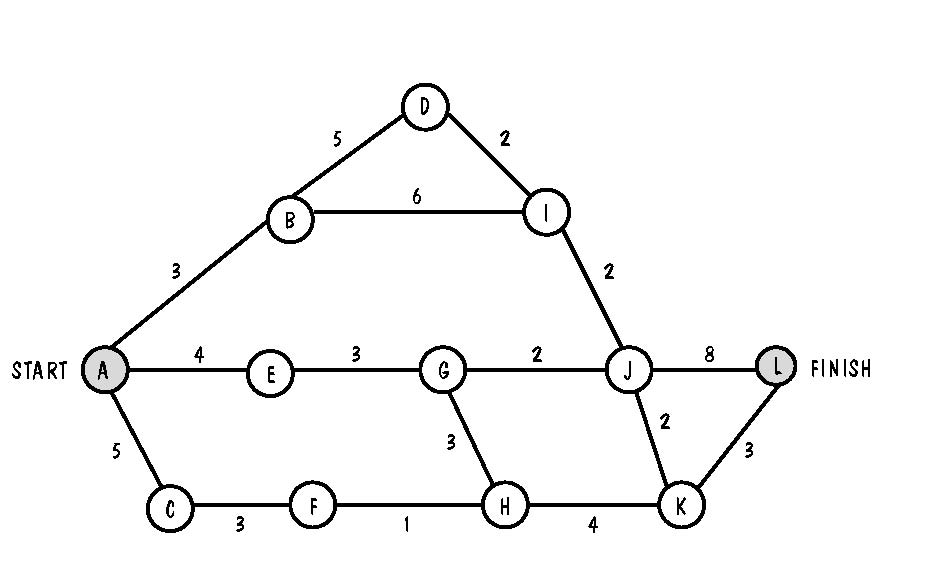求各个路径的总权值，权值最大的即为关键路径 ABDIJL 权值为3+5+2+2+8=20 ABDIJKL 权值为3+5+2+2+2+3=17 ABIJL 权值为19 ABIJKL 权值为16 AEGJL 权值为17 AEGJKL 权值为14 AEGHKL 权值为17 ACFHKL 权值为16 由此可知关键路径为ABDIJL。 回溯求出最早，最晚开始时间和差值 **！！！！：对于关键路径上的活动最早最晚开始时间的差值始终为0；最晚开始时间=后驱节点对应的时间-活动时间 （如果后驱节点对应多个时间，选取最小的那个）**最早开始时间=max{到达前驱结点的路径权值}+1 （这个加1是为什么呢？举个例子，一个工程的前半部分需要20天，从月初的1号开始，在20号正好完成，所以后半部分工程从21号开始） e.p. 活动KL的的前驱节点为K， 最晚开始时间=（20+1）-3=18 （此处加1意义同上，但在计算最晚开始时间时只在最后活动加1，其他活动不必再加1，考虑考虑，这是符合常理的） 最早开始时间=max{c(ABDIJ),c(ABIJ),c(AEGJ),c(AEGH),c(ACFH)}+1=15 所以， KL: Precursor{K} , Earliest start Time:15, latest Start Time:18 Slacktime:3;
在计算一个HK： 通过计算KL我们知道K对应的最晚开始 时间为18 最晚开始时间=18-4=14 最早开始时间=11（方法同上）
所以此题所有答案如下：
AB: Precursor{A} , Earliest start Time:1, latest Start Time:1 Slacktime:0; BD: Precursor{B} , Earliest start Time:4, latest Start Time:4 Slacktime:0; DI: Precursor{D} , Earliest start Time:9, latest Start Time:9 Slacktime:0; BI: Precursor{B} , Earliest start Time:4, latest Start Time:5 Slacktime:1; AE: Precursor{A} , Earliest start Time:1, latest Start Time:5 Slacktime:3; EG: Precursor{E} , Earliest start Time:5, latest Start Time:8 Slacktime:3; GJ: Precursor{G} , Earliest start Time:8, latest Start Time:11 Slacktime:3; GH: Precursor{G} , Earliest start Time:8, latest Start Time:11 Slacktime:3; IJ: Precursor{I} , Earliest start Time:11, latest Start Time:11 Slacktime:0; AC: Precursor{A} , Earliest start Time:1, latest Start Time:5 Slacktime:4; CF: Precursor{A} , Earliest start Time:6, latest Start Time:10 Slacktime:4; FH: Precursor{F} , Earliest start Time:9, latest Start Time:13 Slacktime:4; HK: Precursor{H} , Earliest start Time:11, latest Start Time:14 Slacktime:3; JK: Precursor{J} , Earliest start Time:13, latest Start Time:16 Slacktime:3; JL: Precursor{J} , Earliest start Time:13, latest Start Time:13 Slacktime:0; KL: Precursor{K} , Earliest start Time:15, latest Start Time:18 Slacktime:3;
另外需要说明一点的是，在计算最晚开始时间时，如果后驱节点对应多个时间，选取最小的那个。 例如，IJ J对应的最晚开始时间分别出现在JK和JL,选取小的那个13。
注：复习软件工程时，在博客园看见的文章，获益匪浅，原文链接如下
https://www.cnblogs.com/wonpangnew/p/5171295.html


展开全文• 关键路径法将项目分解成为多个独立的活动并确定每个活动的工期，然后用逻辑关系（结束-开始、结束-结束、开始-开始开始结束）将活动连接，从而能够计算项目的工期、各个活动时间特点（最晚时间...软件工程
• 仿滴滴时间选择器，最晚出发时间开始结束时间。过滤时间。自定义时间选择器，效果图https://mp.csdn.net/console/editor/html/109441069
• Element UI 结束时间开始时间 <el-form-item label="开始结束时间"> <el-time-picker v-model="form.startTime" @change="startChange" value="HH:mm:ss" value-format="HH:mm:ss"...
• 场景：一张定单有多个环节，一个环节可能出现多次，计算每个环节从派发到完成的时间1.介绍 LAST_VALUE 和 FIRST_VALUE 函数2.sql实战示例 1.介绍 LAST_VALUE 和 FIRST_VALUE 函数 函数的作用恰如其名，取出首尾记录...oracle
• 简而言之，就是earliest time和latest time的定义，两者都是基于Activity的，就是字面意思，但是都是（早／最晚开始时间 还定义了 ;">关键Activity ，即earliest time == latest time的Activity ...
• 开始时间不能于结束时间 背景： 项目中有个需求是开始时间不能于结束时间，有段时间不接触element ui了，给忘记了，网 上找了很多案例，但是每一个是我想要的，于是自己操作一番，记下来方便以后借鉴。 ...vue.js
• 先贴页面代码： &lt;li class="form-group " style="width:31%;position:relative;"&gt; &lt;label class="...开始时间:&lt;/label&gt; &lt;div
• mysql导入打卡记录，查询员工每天早上班时间最晚下班时间
• 工作M的开始时间为第23天（23+5=28，第28天要开始另外的工作了，所以最晚必须第23天开始），开始时间为第16天，所以总时差有7天。 早完成工作M的时间是第21天，下次项目开始的早是第25天，所以自由时差...
• 方法1 select * from 表 where openid='****' order by (fwsj - to_timestamp(to_char(fwsj,'YYYY-MM-DD'),'YYYY...取时间 倒序排序取最晚时间 方法2： select * from 表 where openid='***' order by (extract(...SQL postgresql
• 关键路径：开始时间 计算方式：每个节点找它到下个节点的最大路径 具有最大路径长度的路径为关键路径。因为你得保证每个人都到场才能去看电影嘛，那你肯定得等到最后一个人到了才能走。图中就是v...算法
• 夏南 ...21岁开始练字？ 23岁如何开始练字？ 23岁想开始练习书法，还来得急吗？应该怎么练字呢? 25岁写字很差，如何练书法？想写成南笙那样就可以 作为快三十岁的写得一手丑字的人该怎么去字体
• 纽约时间比加州时间早三个小时， New York is 3 hours ahead of California, 但加州时间并没有变慢。 but it does not make California slow. 有人22岁就毕业了， Someone graduated at the age of 22, 但等了五年才...
• ## 30岁开始学编程晚吗？

万次阅读 多人点赞 2020-04-09 09:52:19
之前是干别的，但有点编程的基础，最近想转行程序员，不知道，你能不能给我一些建议，或者说有没有一些攻略可以参考。 这是上个月一个读者小郑私信我的问题，我一直置顶着，想不清楚该怎么回答他。最近又遇到...算法 程序员 编程
• 今天在做需求的时候，其中有一个环节需要获取当前时间，并且根据当前时间获取当前时间的开始时间（00：00：00）和结束时间（23：59：59）。 这时候可以使用jdk自带的工具类DateUtil来实现。 首先new一个Date对象。 ...
• timeout_express字段可以理解为，用户输入支付密码/或签约支付发起扣费，支付宝开始进行轮询用户可用支付方式的开始(好像支付宝收银台的话，如果用户欠费，会直接给打回来) 说一下，出现问题的两个场景 场景一： ...java ios android 小程序
• 开始我是这样写的：起始日期" onFocus="WdatePicker({maxDate:'#F{$dp.$D(\'d4312\')}'|| '%y-%M-%d'})" /><input id="d4312" class=datepicker angularjs
• ## 人生永远没有太晚的开始

千次阅读 多人点赞 2020-05-02 00:01:22
摩西奶奶说：你愿意做的那件事，才是你真正的天赋所在。　我的童年是在奶奶家渡过的，小学二年级时才回到父母身边。在我的印象里，奶奶家是一个逼仄的小平房。屋子从地上陷下去一块，一脚迈进...
• 子验证者 将字幕文件从英语翻译成其他语言时，有时必须更改时间。 该程序通过报告与计时相关的错误来验证完成的翻译字幕文件（例如，字幕在同一时间运行，或者开始时间晚于结束时间）Java
• 数据结构AOE网关键路径问题，不算活动差，直接看事件早差，差为0的就是关键路径，这样做对么？ 数据结构AOE网关键路径问题，不算活动差，直接看事件早差，差为0的就是关键路径，这样做对么？我做了几道......